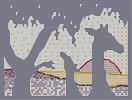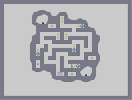### Kabrizzly BearHover over the thumbnail for a full-size version.

Author BE_nSPIRED author:be_nspired bear grizzly inspired kabrizzly n-art rated 2009-11-26 2009-11-26 4 by 9 people. \$Kabrizzly Bear#BE_nSPIRED#kabrizzly#00000000000000000000000000000000000000000000000000000000000000000000000000000000000000000000000000000000000000000000000000000000000000000000000000000000000000000000000000000000000000000000000000000000000000000000000000000000000000000000000000000000000000000000000000000000000000000000000000000000000000000000000000000000000000000000000000000000000000000000000000000000000000000000000000000000000000000000000000000000000000000000000000000000000000000000000000000000000000000000000000000000000000000000000000000000000000000000000000000000000000000000000000000000000000000000000000000000000000000000000000000000000000000000000000000000000000000000000000000000000000000000000000000000000000000000000000000000000000000|10^118,468!10^123,465!10^123,463!10^125,463!10^126,461!10^127,459!10^130,451!10^130,450!10^130,444!10^131,440!10^132,435!10^134,430!10^137,424!10^139,416!10^142,410!10^144,408!10^146,401!10^146,398!10^146,390!10^146,384!10^146,380!10^146,372!10^146,366!10^146,359!10^146,345!10^146,345!10^148,345!10^150,337!10^152,332!10^156,322!10^158,316!10^160,305!10^162,291!10^139,313!10^141,318!10^143,326!10^144,336!10^144,339!10^147,347!10^147,355!10^147,366!10^187,136!10^187,140!10^187,142!10^178,146!10^176,147!10^176,147!10^174,150!10^170,151!10^164,156!10^163,160!10^163,168!10^160,171!10^155,174!10^151,179!10^151,183!10^151,188!10^151,192!10^149,195!10^149,201!10^149,206!10^149,212!10^149,217!10^149,225!10^149,229!10^149,233!10^149,239!10^152,243!10^152,247!10^152,249!10^156,251!10^156,252!10^151,258!10^153,267!10^154,271!10^158,275!10^159,278!10^161,282!10^162,286!10^162,292!10^162,299!10^162,304!10^160,311!10^156,316!10^180,276!10^180,280!10^180,289!10^178,297!10^178,308!10^178,308!10^178,300!10^180,292!10^186,297!10^186,304!10^188,309!10^189,311!10^192,315!10^198,321!10^201,325!10^204,329!10^208,331!10^213,337!10^219,339!10^223,341!10^228,345!10^231,346!10^236,347!10^240,351!10^243,353!10^164,463!10^169,465!10^173,469!10^175,470!10^165,442!10^168,446!10^176,452!10^179,457!10^184,459!10^184,468!10^189,470!10^196,474!10^201,478!10^196,468!10^201,471!10^180,444!10^187,449!10^197,454!10^203,459!10^208,462!10^211,465!10^197,457!10^191,452!10^184,448!10^277,392!10^281,394!10^285,398!10^287,401!10^289,404!10^291,409!10^293,413!10^295,418!10^298,424!10^301,431!10^303,440!10^305,443!10^306,450!10^211,364!10^215,369!10^221,372!10^226,377!10^228,379!10^229,381!10^231,384!10^233,388!10^236,391!10^239,395!10^242,400!10^245,402!10^247,406!10^251,412!10^253,414!10^255,417!10^257,421!10^259,425!10^261,430!10^262,432!10^265,435!10^267,438!10^273,443!10^277,445!10^281,446!10^284,448!10^292,449!10^191,371!10^196,371!10^202,374!10^205,377!10^208,379!10^211,382!10^214,385!10^218,387!10^222,389!10^226,392!10^229,393!10^231,394!10^196,387!10^201,391!10^208,395!10^212,398!10^216,401!10^218,403!10^220,405!10^224,408!10^226,411!10^231,414!10^197,411!10^205,412!10^207,414!10^210,415!10^213,417!10^216,418!10^221,419!10^223,421!10^225,423!10^230,426!10^235,431!10^204,441!10^207,443!10^213,447!10^216,450!10^220,452!10^225,456!10^232,461!10^237,465!10^241,470!10^245,473!10^249,476!10^252,478!10^257,479!10^262,480!10^269,480!10^272,480!10^280,480!10^287,480!10^291,481!10^297,483!10^305,485!10^311,486!10^318,486!10^319,486!10^324,488!10^328,490!10^330,491!10^334,491!10^337,492!10^340,492!10^340,492!10^345,493!10^347,493!10^352,494!10^294,453!10^299,457!10^303,459!10^307,462!10^311,465!10^318,468!10^324,471!10^327,474!10^331,477!10^335,480!10^227,455!10^229,456!10^236,458!10^239,458!10^245,459!10^248,460!10^253,462!10^255,462!10^219,436!10^221,437!10^225,441!10^231,443!10^234,445!10^239,446!10^245,447!10^250,451!10^253,453!10^259,457!10^254,441!10^260,441!10^265,441!10^200,439!10^278,462!10^279,462!10^285,464!10^287,466!10^291,468!10^295,470!10^297,473!10^299,476!10^303,478!10^307,481!10^308,447!10^310,448!10^313,450!10^265,424!10^272,431!10^278,434!10^284,436!10^214,430!10^225,260!10^226,260!10^233,261!10^236,262!10^238,262!10^242,262!10^245,263!10^246,266!10^246,268!10^242,274!10^237,276!10^235,279!10^232,281!10^251,281!10^252,281!10^250,286!10^248,288!10^245,293!10^245,299!10^252,294!10^256,294!10^261,296!10^265,301!10^273,301!10^258,305!10^256,310!10^256,318!10^184,212!10^182,216!10^182,217!10^177,220!10^183,222!10^186,225!10^188,226!10^184,231!10^182,234!10^199,151!10^196,155!10^196,160!10^192,165!10^191,183!10^191,189!10^191,192!10^187,195!10^204,189!10^196,195!10^191,197!10^191,197!10^191,131!10^191,128!10^186,122!10^183,120!10^183,118!10^181,115!10^181,108!10^181,102!10^181,95!10^179,88!10^181,79!10^185,77!10^191,71!10^194,71!10^200,71!10^208,72!10^212,75!10^214,77!10^216,81!10^193,78!10^186,91!10^186,99!10^186,108!10^194,84!10^208,72!10^197,85!10^187,106!10^195,102!10^205,85!10^207,80!10^195,94!10^192,109!10^213,90!10^211,88!10^205,103!10^193,118!10^199,94!10^203,94!10^245,91!10^240,90!10^233,90!10^243,107!10^237,109!10^233,113!10^228,115!10^225,121!10^231,130!10^227,131!10^221,134!10^230,140!10^230,143!10^224,147!10^217,150!10^235,162!10^232,165!10^225,169!10^233,165!10^235,169!10^239,174!10^239,182!10^232,186!10^223,192!10^267,171!10^260,174!10^256,179!10^257,183!10^255,188!10^246,193!10^238,197!10^238,199!10^235,207!10^235,215!10^261,189!10^201,208!10^232,216!10^228,216!10^231,221!10^232,225!10^237,229!10^239,229!10^243,229!10^246,220!10^258,225!10^269,232!10^267,233!10^265,238!10^274,247!10^278,250!10^287,235!10^287,249!10^287,253!10^288,246!10^288,239!10^298,248!10^298,254!10^300,259!10^300,261!10^304,265!10^312,257!10^248,432!10^250,432!10^253,432!10^278,298!10^282,298!10^286,304!10^288,306!10^290,307!10^292,309!10^294,312!10^296,315!10^300,317!10^301,321!10^303,325!10^304,326!10^308,329!10^312,332!10^314,333!10^209,70!10^213,70!10^214,68!10^217,66!10^220,62!10^223,59!10^225,59!10^229,57!10^234,55!10^236,55!10^240,55!10^241,53!10^243,53!10^259,43!10^252,48!10^245,51!10^241,52!10^267,49!10^263,45!10^267,45!10^273,45!10^278,45!10^284,43!10^289,43!10^299,43!10^302,43!10^309,45!10^316,46!10^325,47!10^332,49!10^334,49!10^335,49!10^411,46!10^406,46!10^400,46!10^397,41!10^393,39!10^388,37!10^381,37!10^374,37!10^368,35!10^363,35!10^356,36!10^348,39!10^343,40!10^338,40!10^333,41!10^327,41!10^323,42!10^318,43!10^310,43!10^427,61!10^430,66!10^430,69!10^434,73!10^436,76!10^440,79!10^442,87!10^446,88!10^449,91!10^452,94!10^458,101!10^460,103!10^463,106!10^466,108!10^474,112!10^478,117!10^482,123!10^485,126!10^486,130!10^476,130!10^476,131!10^481,136!10^489,144!10^490,145!10^482,139!10^492,150!10^498,157!10^498,160!10^502,166!10^504,171!10^504,175!10^510,180!10^515,185!10^518,189!10^428,53!10^433,53!10^440,56!10^446,65!10^449,71!10^449,77!10^445,75!10^437,63!10^430,56!10^437,68!10^443,78!10^451,83!10^451,76!10^451,66!10^446,56!10^433,52!10^416,54!10^419,53!10^315,99!10^335,99!10^337,96!10^339,96!10^342,90!10^335,92!10^333,90!10^331,89!10^324,103!10^331,102!10^320,102!10^323,88!10^322,88!10^313,96!10^313,96!10^316,92!10^369,48!10^371,50!10^374,52!10^380,55!10^383,57!10^388,59!10^391,60!10^391,64!10^388,65!10^385,68!10^390,74!10^391,75!10^394,75!10^382,73!10^377,71!10^374,69!10^370,67!10^366,67!10^361,68!10^356,71!10^349,67!10^352,70!10^353,74!10^353,76!10^353,81!10^312,130!10^317,131!10^319,131!10^326,131!10^329,129!10^332,129!10^338,129!10^338,129!10^340,129!10^307,148!10^308,150!10^312,155!10^314,158!10^318,160!10^320,162!10^303,163!10^299,175!10^318,223!10^319,227!10^323,230!10^325,233!10^323,189!10^323,192!10^323,195!10^325,201!10^327,205!10^329,207!10^331,209!10^335,213!10^337,215!10^513,186!10^513,187!10^513,193!10^513,198!10^514,203!10^517,211!10^519,217!10^519,226!10^519,230!10^519,240!10^514,255!10^511,257!10^509,259!10^522,239!10^509,248!10^513,249!10^502,250!10^500,259!10^498,260!10^497,262!10^494,264!10^490,269!10^487,273!10^485,274!10^483,280!10^479,290!10^479,291!10^475,296!10^471,301!10^467,306!10^461,312!10^459,320!10^452,328!10^452,334!10^452,339!10^452,349!10^415,419!10^415,417!10^415,411!10^416,404!10^420,396!10^424,393!10^430,383!10^430,381!10^432,378!10^434,370!10^438,358!10^438,355!10^438,349!10^436,366!10^525,211!10^523,211!10^528,211!10^532,211!10^532,212!10^536,215!10^540,217!10^542,218!10^546,219!10^548,219!10^549,219!10^551,219!10^547,228!10^546,229!10^549,234!10^556,235!10^560,237!10^564,238!10^568,240!10^574,243!10^564,247!10^562,251!10^571,253!10^577,254!10^582,254!10^585,254!10^558,262!10^558,263!10^558,266!10^558,258!10^569,261!10^574,263!10^580,268!10^586,272!10^590,275!10^596,276!10^592,282!10^601,286!10^606,287!10^611,291!10^615,295!10^618,297!10^621,300!10^624,301!10^628,303!10^632,307!10^636,317!10^638,318!10^645,321!10^650,323!10^657,327!10^660,328!10^666,330!10^670,334!10^672,339!10^678,348!10^681,352!10^682,354!10^684,359!10^687,366!10^689,368!10^692,372!10^692,375!10^693,379!10^696,383!10^700,386!10^701,391!10^705,398!10^707,403!10^709,409!10^712,417!10^715,429!10^716,430!10^719,436!10^721,442!10^722,448!10^566,283!10^567,289!10^576,291!10^579,312!10^580,312!10^590,312!10^593,313!10^596,313!10^593,439!10^593,439!10^602,444!10^605,447!10^613,451!10^616,455!10^618,457!10^621,461!10^623,464!10^625,466!10^628,437!10^628,442!10^635,447!10^637,452!10^639,456!10^640,460!10^641,464!10^645,467!10^648,469!10^652,469!10^635,440!10^640,441!10^645,445!10^650,447!10^654,449!10^657,451!10^663,453!10^668,454!10^659,434!10^662,437!10^673,439!10^680,443!10^684,445!10^689,447!10^667,436!10^630,411!10^635,412!10^644,415!10^648,416!10^648,416!10^650,418!10^652,419!10^658,420!10^664,422!10^666,423!10^670,425!10^672,427!10^675,428!10^679,429!10^682,432!10^684,434!10^688,436!10^693,438!10^698,441!10^701,443!10^703,445!10^706,446!10^666,383!10^666,383!10^666,393!10^666,396!10^666,402!10^666,409!10^669,416!10^672,419!10^652,391!10^657,397!10^671,406!10^672,409!10^682,416!10^685,419!10^692,422!10^686,382!10^686,392!10^689,401!10^695,405!10^712,442!10^712,447!10^712,454!10^712,456!10^713,460!10^714,468!10^715,479!10^717,484!10^715,493!10^713,494!10^710,497!10^699,502!10^696,505!10^696,505!10^688,509!10^683,512!10^678,515!10^669,518!10^667,519!10^664,522!10^656,524!10^651,531!10^648,534!10^641,541!10^639,541!10^648,533!10^634,543!10^629,548!10^621,551!10^612,554!10^609,556!10^604,558!10^687,485!10^684,491!10^674,496!10^671,499!10^665,502!10^661,506!10^650,513!10^640,519!10^631,530!10^627,533!10^602,473!10^602,476!10^607,478!10^611,481!10^617,481!10^624,481!10^602,492!10^603,493!10^608,496!10^608,497!10^543,495!10^543,498!10^541,503!10^538,505!10^535,506!10^533,510!10^529,511!10^406,563!10^406,570!10^407,575!10^448,552!10^446,557!10^440,562!10^440,570!10^435,570!10^431,572!10^425,573!10^541,538!10^541,540!10^539,548!10^537,550!10^533,551!10^558,564!10^554,561!10^550,557!10^546,563!10^543,569!10^539,567!10^529,573!10^523,577!10^596,558!10^589,561!10^583,563!10^574,565!10^627,533!10^615,537!10^611,542!10^607,545!10^601,549!10^704,494!10^697,497!10^691,501!10^442,355!10^442,358!10^440,367!10^440,372!10^438,377!10^438,384!10^438,386!10^438,392!10^436,398!10^436,405!10^434,410!10^430,418!10^430,424!10^430,430!10^426,433!10^424,436!10^417,443!10^417,450!10^417,452!10^413,457!10^408,471!10^494,312!10^490,317!10^488,324!10^486,332!10^484,336!10^482,341!10^478,348!10^474,351!10^477,348!10^486,341!10^494,341!10^496,339!10^505,353!10^497,353!10^491,353!10^489,358!10^484,363!10^484,363!10^490,366!10^494,364!10^494,369!10^494,373!10^494,377!10^490,378!10^550,378!10^545,381!10^539,385!10^535,388!10^535,392!10^539,396!10^546,396!10^538,396!10^536,400!10^530,406!10^530,408!10^533,417!10^535,417!10^533,415!10^528,418!10^528,420!10^524,425!10^524,432!10^525,441!10^527,441!10^521,439!10^516,439!10^519,421!10^514,421!10^509,419!10^505,413!10^506,412!10^514,396!10^507,400!10^504,405!10^500,411!10^500,415!10^493,423!10^493,425!10^493,428!10^493,433!10^489,437!10^485,439!10^485,442!10^482,445!10^474,451!10^469,454!10^466,454!10^464,455!10^459,459!10^455,463!10^450,466!10^445,468!10^446,381!10^446,390!10^442,400!10^440,403!10^440,408!10^440,412!10^440,417!10^440,421!10^437,426!10^433,436!10^433,449!10^433,456!10^431,459!10^476,475!10^478,478!10^479,486!10^480,488!10^480,495!10^478,500!10^475,503!10^471,512!10^459,523!10^417,554!10^417,553!10^417,543!10^417,536!10^420,533!10^426,525!10^417,522!10^425,522!10^422,513!10^416,511!10^418,505!10^439,496!10^447,490!10^436,497!10^421,503!10^428,499!10^431,499!10^371,491!10^378,494!10^382,497!10^385,501!10^406,501!10^402,501!10^412,492!10^417,487!10^426,483!10^430,483!10^434,479!10^431,479!10^427,472!10^427,472!10^461,395!10^459,404!10^459,407!10^459,415!10^459,420!10^459,425!10^459,433!10^459,434!10^462,434!10^465,431!10^468,424!10^478,418!10^479,418!10^470,423!10^474,382!10^466,383!10^466,385!10^453,417!10^449,427!10^445,432!10^445,437!10^445,444!10^445,454!10^441,456!10^458,427!10^443,436!10^441,449!10^441,458!10^436,464!10^444,474!10^446,474!10^394,96!10^396,96!10^400,96!10^402,94!10^409,94!10^413,95!10^413,97!10^404,97!10^402,97!10^398,97!10^388,114!10^391,115!10^395,115!10^413,112!10^420,110!10^407,131!10^414,145!10^414,147!10^411,151!10^409,152!10^409,153!10^407,156!10^404,156!10^403,156!10^398,158!10^397,158!10^396,159!10^390,160!10^388,161!10^383,161!10^382,161!10^378,161!10^374,164!10^371,171!10^368,172!10^364,175!10^362,176!10^359,176!10^356,176!10^351,176!10^343,180!10^345,183!10^350,186!10^353,187!10^353,190!10^353,197!10^351,198!10^341,199!10^385,168!10^377,169!10^382,169!10^391,169!10^395,169!10^401,169!10^404,169!10^411,169!10^417,170!10^419,170!10^429,170!10^432,170!10^433,170!10^436,170!10^436,170!10^436,168!10^436,165!10^432,158!10^430,158!10^422,154!10^417,145!10^419,150!10^424,167!10^421,163!10^419,163!10^414,161!10^425,84!10^429,84!10^430,87!10^426,88!10^421,84!10^423,97!10^426,101!10^432,104!10^458,111!10^458,114!10^456,119!10^454,124!10^452,126!10^450,129!10^446,135!10^444,140!10^446,140!10^453,140!10^456,142!10^457,150!10^457,150!10^457,152!10^457,159!10^459,155!10^459,150!10^459,141!10^451,169!10^460,169!10^460,169!10^465,170!10^468,171!10^471,173!10^471,178!10^471,182!10^471,188!10^471,191!10^471,193!10^471,201!10^471,207!10^471,211!10^471,216!10^467,214!10^463,203!10^460,196!10^456,189!10^453,184!10^449,181!10^447,188!10^447,198!10^447,201!10^447,207!10^447,215!10^447,217!10^447,225!10^437,237!10^433,238!10^442,231!10^449,226!10^455,224!10^474,219!10^467,223!10^463,229!10^441,191!10^441,200!10^441,205!10^430,201!10^430,206!10^430,210!10^423,208!10^421,211!10^413,208!10^413,209!10^393,215!10^321,275!10^325,275!10^328,280!10^329,285!10^335,268!10^339,271!10^341,276!10^345,282!10^353,280!10^359,282!10^365,236!10^367,247!10^370,252!10^374,257!10^371,225!10^376,230!10^380,236!10^382,241!10^384,246!10^386,248!10^397,237!10^398,237!10^400,242!10^409,239!10^469,235!10^464,237!10^464,241!10^462,243!10^462,250!10^460,255!10^460,264!10^460,271!10^454,277!10^447,283!10^432,288!10^435,302!10^433,292!10^436,300!10^438,306!10^440,314!10^436,314!10^428,308!10^421,305!10^412,298!10^404,296!10^410,302!10^414,306!10^419,311!10^421,314!10^413,314!10^404,308!10^394,301!10^386,296!10^382,285!10^382,287!10^386,296!10^389,300!10^393,304!10^399,308!10^406,317!10^409,324!10^409,334!10^400,325!10^395,317!10^388,309!10^385,308!10^381,309!10^384,317!10^385,322!10^385,330!10^385,331!10^386,335!10^388,342!10^389,347!10^391,350!10^392,356!10^394,359!10^396,361!10^396,368!10^398,377!10^398,384!10^398,387!10^365,311!10^365,315!10^367,318!10^368,325!10^369,331!10^371,334!10^373,339!10^375,346!10^379,350!10^383,352!10^384,355!10^447,242!10^434,246!10^434,251!10^424,258!10^417,260!10^417,258!10^419,264!10^432,257!10^443,254!10^445,254!10^443,259!10^433,264!10^433,270!10^430,282!10^426,291!10^434,278!10^450,269!10^439,272!10^441,274!10^322,410!10^322,412!10^326,417!10^327,421!10^328,429!10^330,434!10^334,438!10^338,443!10^341,446!10^304,375!10^311,379!10^312,381!10^302,359!10^311,361!10^319,362!10^320,364!10^326,370!10^328,374!10^332,380!10^336,385!10^344,393!10^348,400!10^349,405!10^351,410!10^351,421!10^351,426!10^353,433!10^353,434!10^351,441!10^351,446!10^352,448!10^354,455!10^356,462!10^358,466!10^325,384!10^325,390!10^326,397!10^330,405!10^332,413!10^334,418!10^314,307!10^319,309!10^330,310!10^323,310!10^331,315!10^339,319!10^355,321!10^357,327!10^359,329!10^331,335!10^335,339!10^337,344!10^337,348!10^339,356!10^341,362!10^343,369!10^345,373!10^349,379!10^351,382!10^357,396!10^361,401!10^370,461!10^370,455!10^368,451!10^366,447!10^366,440!10^364,433!10^364,425!10^364,417!10^364,407!10^370,405!10^370,423!10^370,437!10^370,447!10^349,367!10^356,376!10^360,381!10^366,383!10^373,387!10^381,387!10^386,398!10^386,405!10^387,394!10^377,382!10^377,376!10^373,366!10^366,359!10^361,353!10^354,346!10^347,343!10^341,339!3^708,376!3^706,376!3^705,376!3^708,372!3^713,370!3^717,370!3^718,370!3^722,370!3^729,370!3^736,372!3^737,373!3^740,374!3^745,374!3^747,374!3^752,375!3^754,375!3^760,375!3^765,375!3^770,375!3^702,379!3^166,321!3^162,334!3^158,348!3^158,363!3^156,379!3^156,393!3^154,404!3^147,419!3^141,432!3^140,445!3^132,457!3^186,280!3^189,293!3^195,307!3^206,319!3^218,332!3^230,338!3^243,342!3^166,283!3^164,268!3^158,250!3^158,243!3^164,171!3^157,187!3^157,205!3^157,224!3^261,51!3^246,56!3^231,66!3^224,76!3^217,94!3^211,102!3^205,108!3^195,125!3^181,150!3^225,367!3^234,376!3^242,389!3^249,399!3^261,407!3^267,416!3^275,427!3^288,397!3^301,413!3^305,426!3^315,439!3^447,567!3^455,553!3^466,521!3^477,512!3^488,497!3^481,479!3^530,439!3^525,225!3^527,244!3^516,261!3^497,268!3^488,289!3^476,304!3^468,317!3^459,332!3^448,365!3^498,314!3^496,342!3^513,351!3^539,512!3^549,499!3^487,483!3^462,122!3^463,144!3^477,174!3^479,190!3^479,206!3^479,218!3^475,233!3^470,246!3^465,261!3^460,274!3^444,295!3^448,309!3^420,326!3^395,333!3^398,349!3^404,361!3^405,377!3^378,416!3^377,435!3^378,447!3^294,48!3^282,49!3^313,323!3^303,308!3^291,293!3^269,291!3^255,264!3^243,212!3^266,221!3^247,182!3^234,126!3^315,149!3^330,193!3^424,145!3^433,151!3^379,49!3^395,59!3^142,370!3^139,370!3^134,364!3^131,362!3^130,362!3^129,362!3^124,362!3^120,363!3^117,364!3^114,365!3^111,366!3^105,367!3^102,368!3^99,368!3^95,368!3^90,368!3^86,369!3^85,369!3^82,366!3^79,366!3^75,367!3^70,367!3^67,368!3^64,368!3^60,368!3^45,368!3^43,368!3^35,368!3^29,368!3^24,369!3^21,369!3^52,368!3^46,369!3^36,376!3^21,381!3^17,383!3^33,376!3^42,370!3^53,369!3^39,374!3^28,379!3^21,387!3^66,366!3^56,374!3^44,381!3^29,397!3^70,375!3^53,390!3^46,399!3^34,409!3^89,375!3^61,395!3^45,412!3^29,424!3^112,379!3^95,393!3^69,414!3^27,446!3^106,412!3^68,436!3^37,463!3^723,378!3^719,379!3^715,380!3^710,384!3^710,385!3^740,387!3^730,393!3^716,407!3^765,400!3^746,412!3^729,430!3^762,434!3^735,464!3^127,395!3^103,438!3^324,95!10^570,258# It's nothing special so probably wont change your Dronies results but I'd thought I'd submit anyway.

## Other maps by this authorMirror's Edge Chinese Dragon Tileset Teh Bengal Tiger Teh Humble Zebra Giraffe Don't dance with the Drones...

Pages: (0)

### The thing is, whenever I do a really good n-art (imo)

It always fdes into nothing, like my mona lisa for example.

### lol... that must have taken ages...

shame it doesn't really look like a bear tho :P

or a mose

:/

### "Yeah, looks kinda dimented."

QFE
i don't like the head but the rest is good... 4/5

### It's a bear?!

I thought it was a pack of sleeping wolves :/